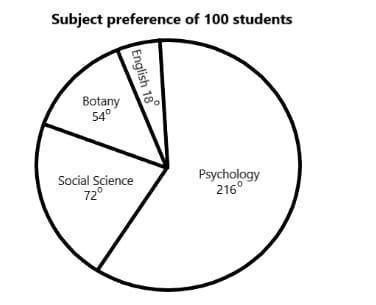The following pie chart shows the result of a survey among 100 students regarding their subject preference.How many students prefer Social Science?

# The following pie chart shows the result of a survey among 100 students regarding their subject preference.How many students prefer Social Science?1. A
20
2. B
30
3. C
40
4. D
10

Fill Out the Form for Expert Academic Guidance!l

+91

Live ClassesBooksTest SeriesSelf Learning

Verify OTP Code (required)

### Solution:

We are given that the pie chart shows a survey among students regarding their subject of preference.
Total number of students =i.estudents
Now, we see that the number of students who prefer social science is given as.studentsstudentsstudents.
Hence,students prefer social science.
Hence, Option (1) is the correct Ans:.

## Related content

 Area of Square Area of Isosceles Triangle Pythagoras Theorem Triangle Formula Perimeter of Triangle Formula Area Formulae Volume of Cone Formula Matrices and Determinants_mathematics Critical Points Solved Examples Type of relations_mathematics+91

Live ClassesBooksTest SeriesSelf Learning

Verify OTP Code (required)